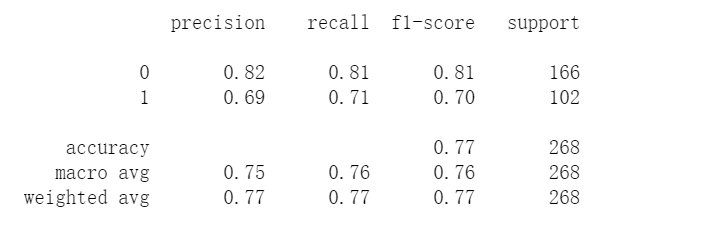• train.csv 用于训练，标记了乘客是否生还的信息
• test.csv  用于测试，没有标记乘客是否生还信息，该数据集需要用模型来预测乘客是否生还

``````# 导入包：
import pandas as pd
import numpy as np
# 读取数据：
# 查看前5行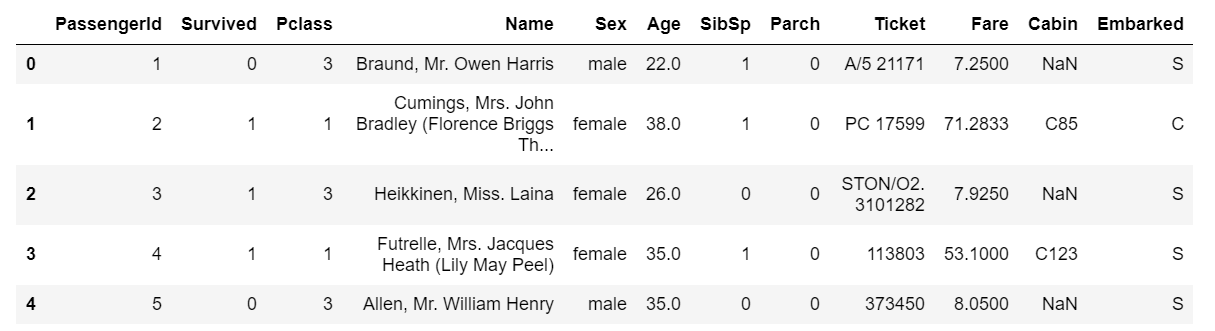• PassengerId：乘客 ID
• Survived：是否生存
• Pclass：客舱等级
• Name：乘客姓名
• Sex：性别
• Age：年龄
• SibSp：在船兄弟姐妹数/配偶数
• Parch：在船父母数/子女数
• Ticket：船票编号
• Fare：船票价格
• Cabin：客舱号
• Embarked：登船港口

``````# 查看数据基本信息
train_data.info()``````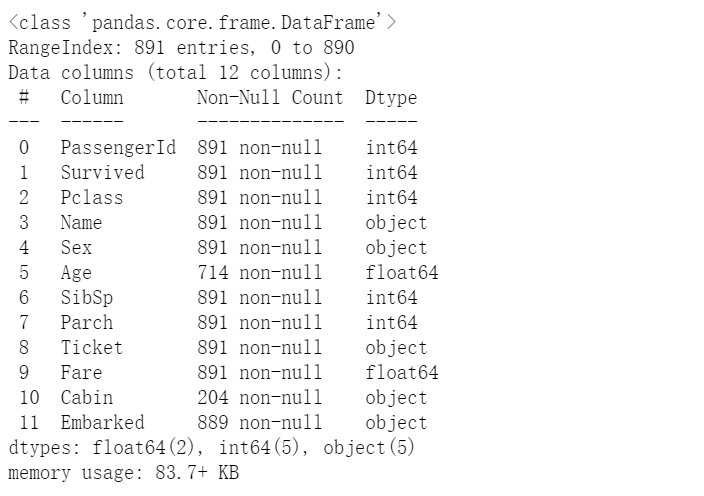``````# 删除无效的数据变量
train_data=train_data.drop(['PassengerId','Name','Ticket'],axis=1)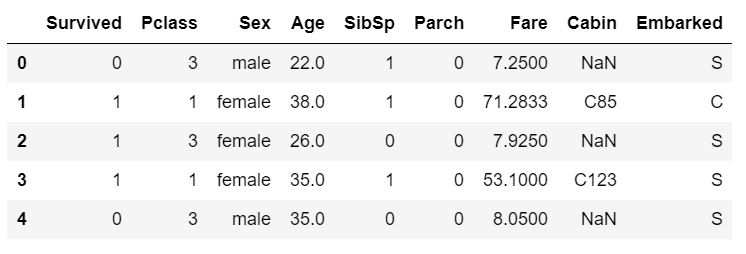• 频数分析：分析类别型变量每一个值出现的频数。
• 描述性统计：对数值型变量的常用统计量进行分析：均值，总和，最小值，最大值，分位数。
• 直方图：对数值型变量进行分段处理后进行频数分析并作图。

1、变量Survived（是否生还）的频数分析及可视化：

``````# 统计频数
Survived_freq=train_data.Survived.value_counts()
print(Survived_freq)
# 统计结果的可视化分析（条形图和饼图）
import matplotlib.pyplot as plt
import seaborn as sns
%matplotlib inline
plt.figure(figsize=(12,5))
plt.subplot(121)
sns.countplot(x='Survived',data=train_data)
plt.title('Count')
plt.subplot(122)
plt.pie(Survived_freq,labels=Survived_freq.index,autopct='%1.0f%%')
plt.title('percent')
plt.show``````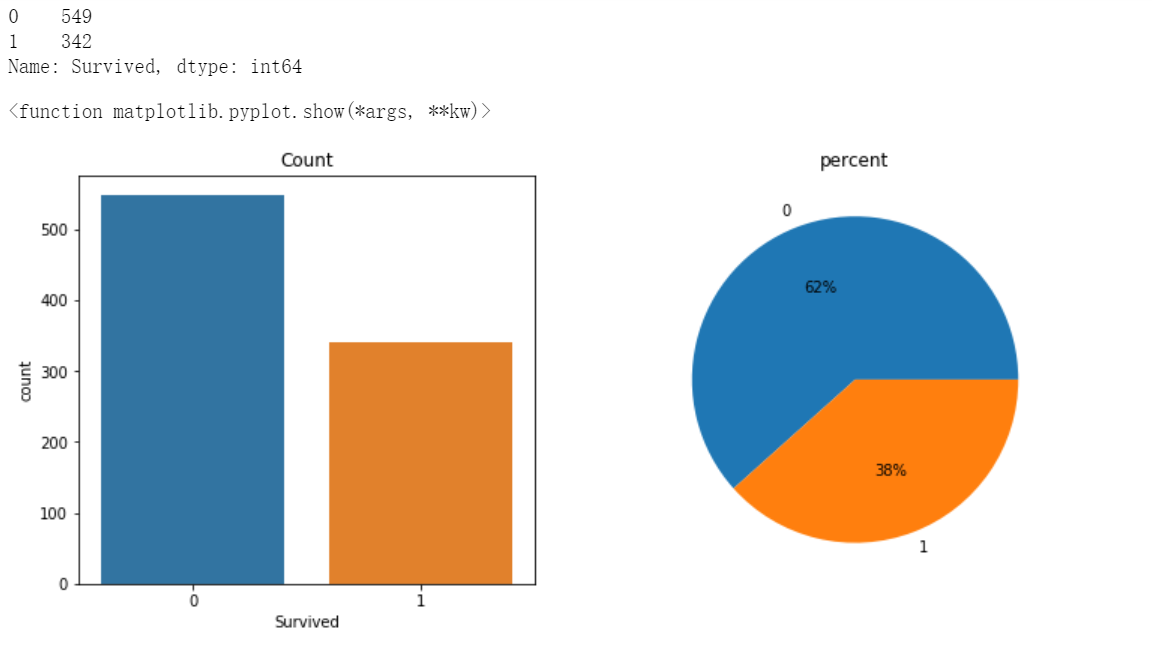2、变量Pclass（客舱等级）的频数分析及可视化：

``````# 统计频数
Pclass_freq=train_data.Pclass.value_counts()
print(Pclass_freq)
# 统计结果的可视化分析（条形图和饼图）
import matplotlib.pyplot as plt
import seaborn as sns
plt.figure(figsize=(12,5))
plt.subplot(121)
sns.countplot(x='Pclass',data=train_data)
plt.title('Count')
plt.subplot(122)
plt.pie(Pclass_freq,labels=Pclass_freq.index,autopct='%1.0f%%')
plt.title('percent')
plt.show()``````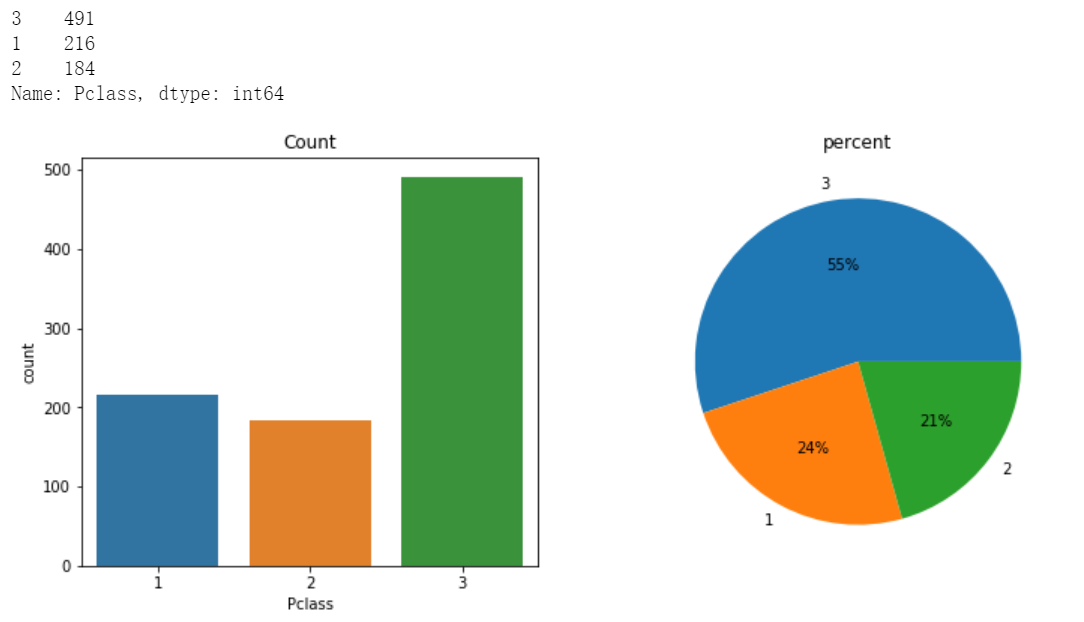3、变量Sex（性别）的频数分析及可视化：

``````# 统计频数
Sex_freq=train_data.Sex.value_counts()
print(Sex_freq)
# 统计结果的可视化分析（条形图和饼图）
import matplotlib.pyplot as plt
import seaborn as sns
plt.figure(figsize=(12,5))
plt.subplot(121)
sns.countplot(x='Sex',data=train_data)
plt.title('Count')
plt.subplot(122)
plt.pie(Sex_freq,labels=Sex_freq.index,autopct='%1.0f%%')
plt.title('percent')
plt.show()``````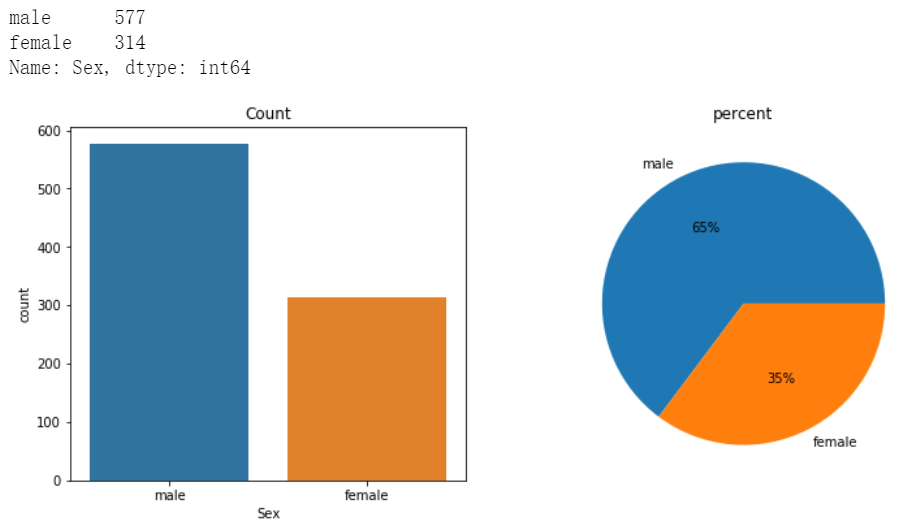从上述分析中可得出训练样本中女性乘客314人，占比35%；男性乘客577人，占65%。

4、变量Embarked（登船港口）的频数分析及可视化：

``````# 统计频数
Embarked_freq=train_data.Embarked.value_counts()
print(Embarked_freq)
# 统计结果的可视化分析（条形图和饼图）
import matplotlib.pyplot as plt
import seaborn as sns
plt.figure(figsize=(12,5))
plt.subplot(121)
sns.countplot(x='Embarked',data=train_data)
plt.title('Count')
plt.subplot(122)
plt.pie(Embarked_freq,labels=Embarked_freq.index,autopct='%1.0f%%')
plt.title('percent')
plt.show()``````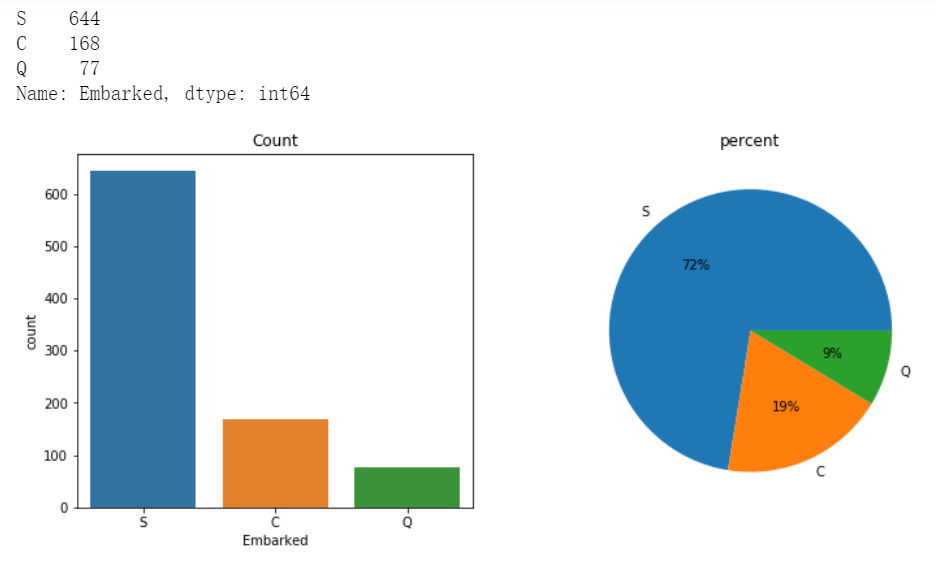``````# Age(年龄)直方图，把Age划分为50个区间绘图直方图，使用hist()
plt.figure(figsize=(20,5))
plt.subplot(221)
train_data['Age'].hist(bins=50)
plt.xlabel('Age')
plt.ylabel('Frequence')
plt.show()``````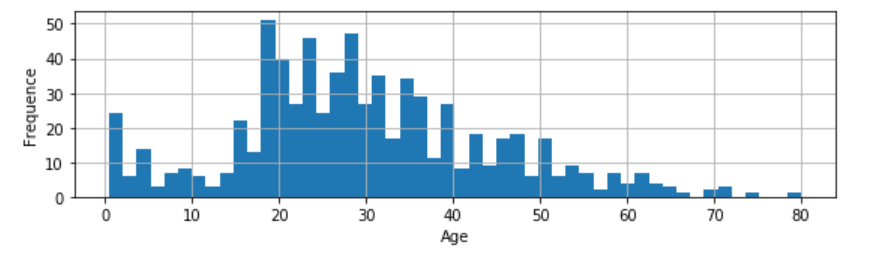``train_data.describe()``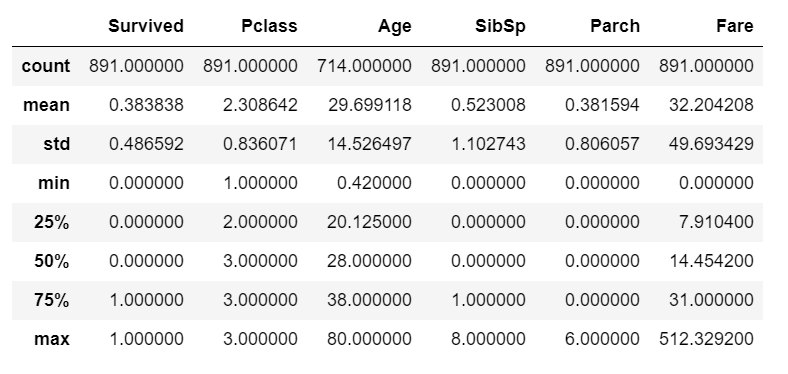• count：非空值频数
• mean：均值
• std：标准差
• min：最小值
• 25%： 25%分位数，又叫下四分位数
• 50%： 50%分位数，又叫中位数(median)
• 75%： 75%分位数，又叫上四分位数
• max：最大值

一般情况下describe()提供的分位数是25%，50%，75%三个位置的分位数，若分位数的精度不够，我们可以通过用qunantile()方法来自定义分位数

``train_data.quantile([0.1,0.2,0.3,0.4,0.5,0.6,0.7,0.8,0.9,1])``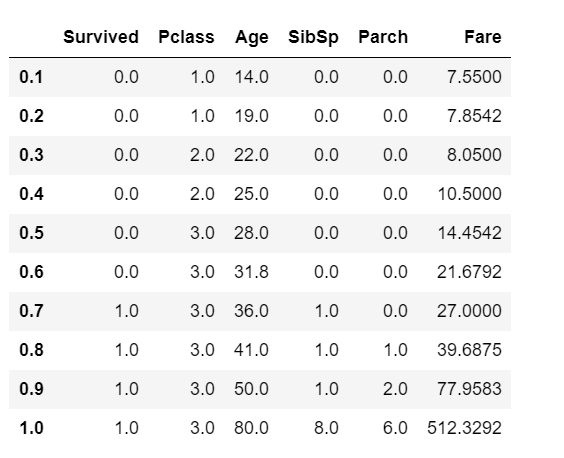• 两个数值型变量之间的相关性方法方法：使用散点图
• 两个类别型变量之间的相关性分析方法：适合用交叉表（crosstab）与堆栈条
• 一个数值型变量，一个类别型变量分析方法：适合用分类汇总(groupby)统计

``````# Age(年龄)和 Fare(船票价格) 两个数值型变量
import  matplotlib.pyplot as plt
plt.scatter(train_data.Age,train_data.Fare,50,'blue')
plt.show()``````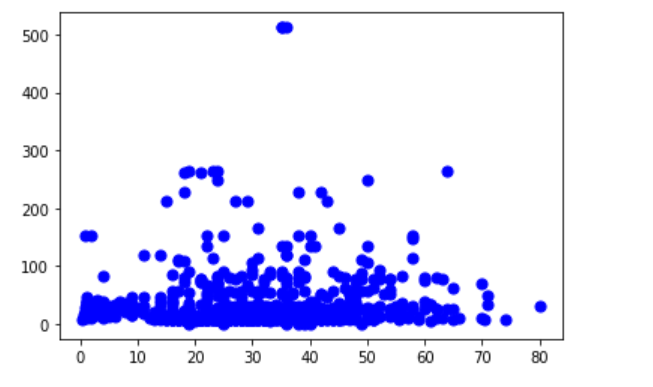``````# Survived VS Pclass交叉表
pd.crosstab(train_data.Survived,train_data.Pclass,margins=True)``````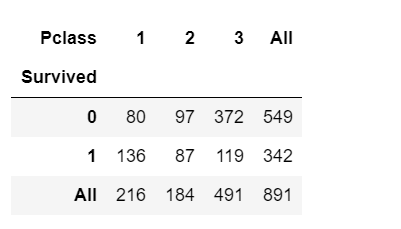``````# Survived VS Pclass 交叉表，单元格显示的是列百分比
pd.crosstab(train_data.Survived,train_data.Pclass).apply(lambda r:r/r.sum(),axis=0)``````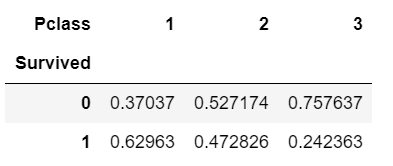• 1等舱的生还率是 62.96%，
• 2等舱的生还率是 47.28%，
• 3等舱的生还率是24.23%

``````# 使用crosstab生成作堆栈条图的数据
# 注：需要去掉margins=True,作图的时候不需要行列汇总的数据
data=pd.crosstab(train_data.Survived,train_data.Pclass)
# 绘制堆栈条图
data.plot(kind='bar',stacked=True)
plt.title('Pclass VS Survived')
plt.ylabel('Frequnce')
plt.show()``````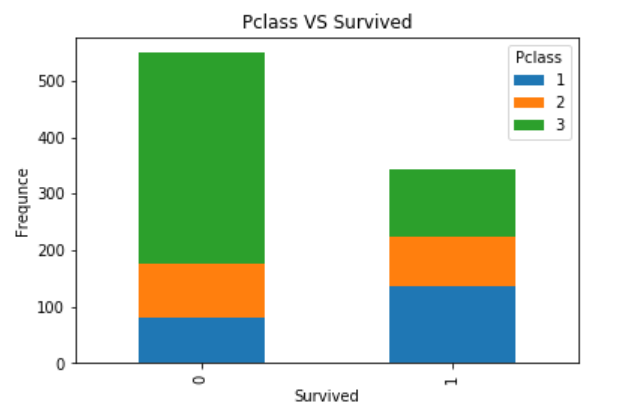``````#方法：对Survived进行汇总，然后统计生还和未生还两类乘客的Age均值差异。
# 先按Survived汇总，求Age和Fare的均值
train_data.groupby('Survived').agg({'Age':'mean','Fare':'mean'})``````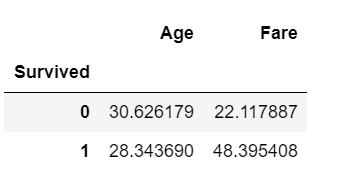``````# 柱形图
data=train_data.groupby('Survived').agg({'Age':'mean','Fare':'mean'})
plt.figure(figsize=(12,5))
plt.subplot(121)
data.Age.plot(kind='bar')
plt.title('Age VS Survived')
plt.ylabel('Average')
plt.subplot(122)
data.Fare.plot(kind='bar')
plt.title('Fare VS Survived')
plt.ylabel('Average')
plt.show()``````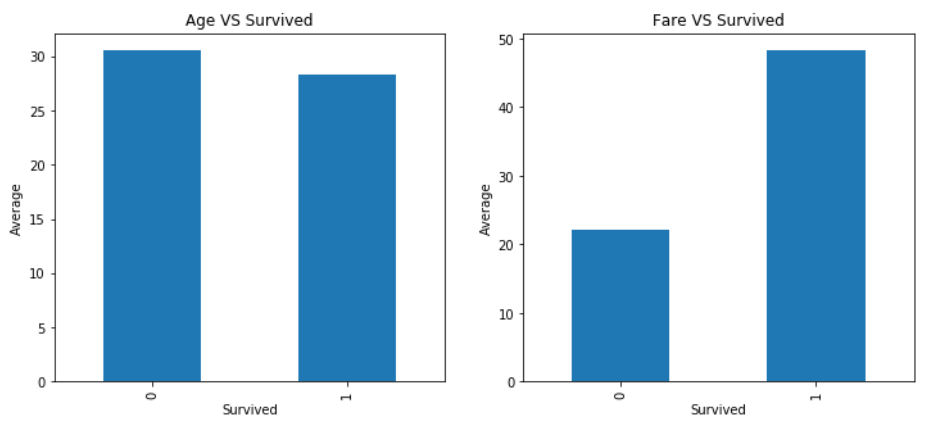• 缺失值的处理
• 对类别型变量的值进行重新编码
• 把连续型变量进行分箱，然后再按照处理类别型变量的方式重新编码
• 对连续型变量进行标准化和归一化处理

``````# 删除整个数据集中任何一个变量有缺失的记录
dropna = train_data.dropna()
dropna.info()``````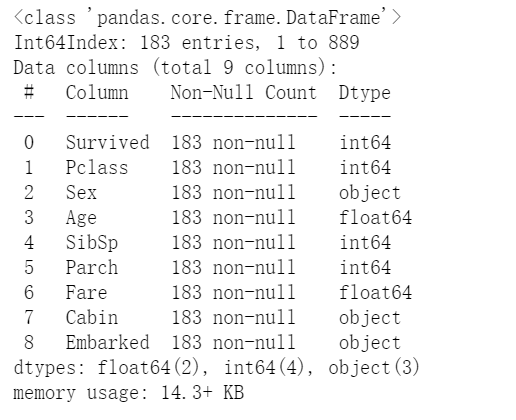``````# 对Age变量删除缺失值，保存为一个新的变量
Age_dropna = train_data[['Age']].dropna()
Age_dropna.info()``````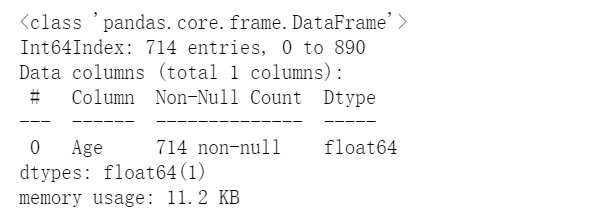``````# 构造新的变量：Age_isna,当Age（年龄）是缺失值的时候Age_isna=1,没有缺失的时候Age_isna=0
train_data['Age_isna']=0
train_data.loc[train_data['Age'].isnull(),'Age_isna']=1
# 对Age_isna进行频数分析，确认Age_isna是否构造正确
train_data['Age_isna'].value_counts()``````替换缺失值（使用均值替换缺失值）

``````# 求均值（使用mean()计算Age的均值）
age_mean=round(train_data['Age'].mean())
# 对年龄缺失值进行均值填充
train_data['Age'].fillna(age_mean,inplace=True)
train_data.Age.describe()``````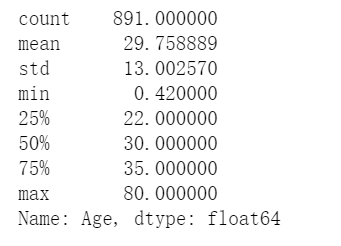``````# 把 Pclass 进行独热编码,prefix 参数设置独热编码后的变量名前缀
Pclass_onehot = pd.get_dummies(train_data.Pclass,prefix='Pclass')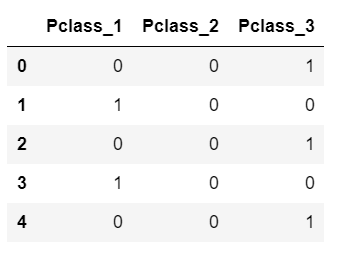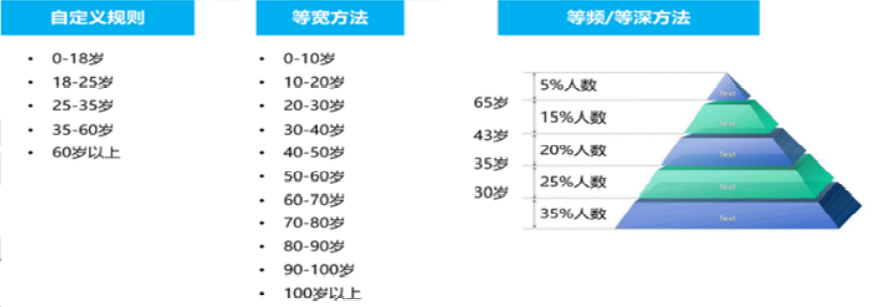``````#对 Age 进行自定义分箱
cut_points = [0,18,25,40,60,100]    # 定义分箱边界值
train_data['Age_bin'] = pd.cut(train_data.Age, bins=cut_points)#分箱后的新变量 Age_bin
train_data['Age_bin'].value_counts() # 分箱后的频数分析
``````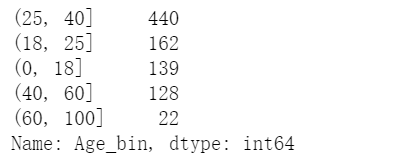``````train_data['Age_wbin'] = pd.cut(train_data.Age,10) # 等宽分箱，分箱数为 10
train_data['Age_wbin'].value_counts()  # 分箱后的频数分析``````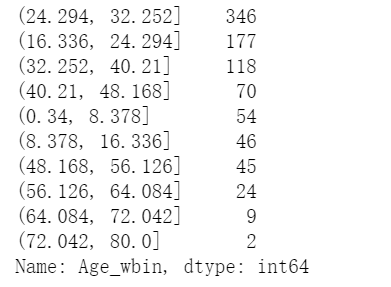``````train_data['Age_dbin'] = pd.qcut(train_data.Age,5)  # 等深分箱，分箱数为 5
train_data['Age_dbin'].value_counts()   # 分箱后的频数分析``````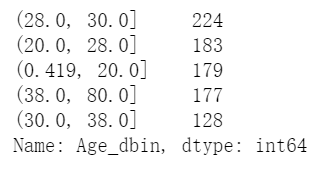``````# 对自定义分箱后的变量进行热度编码
Age_bin_onehot = pd.get_dummies(train_data.Age_bin,prefix='Age_bin')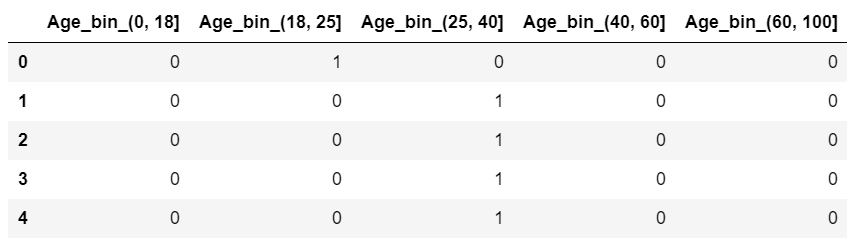•  标准化要做的事情就是把原始的连续型变量转换为均值为0，标准差为1 的变量。使用 sklearn.preprocessing.StandardScaler()来进行均值为 0，标准差为 1 的标准化
• 归一化就是把原始的连续型变量转换为范围在【a-b】之间的变量，常见的a=0，b=1。使用 sklearn.preprocessing.MinMaxScaler()来进行[0-1]之间的归一化

``````# 标准化 先导入包
from sklearn import preprocessing
# 对Age标准化处理后保存为原数据对象中的新变量：Age_std
train_data['Age_std']=preprocessing.scale(train_data[['Age']])
# 标准后的数据
train_data``````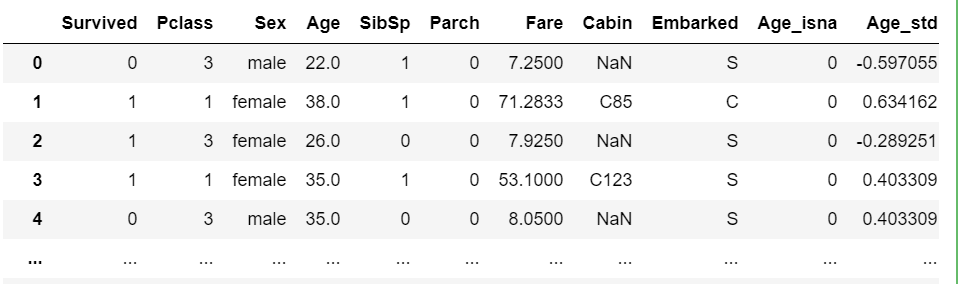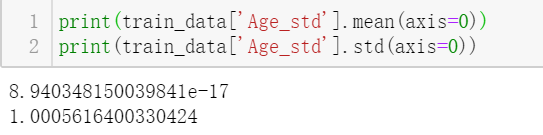``````from sklearn import preprocessing
minmaxscaler=preprocessing.MinMaxScaler()   # 获取最大值和最小值
minmaxscaler.fit(train_data[['Age']])
# Age 归一化处理后保存为新变量 Age_normal
train_data['Age_normal']=minmaxscaler.transform(train_data[['Age']])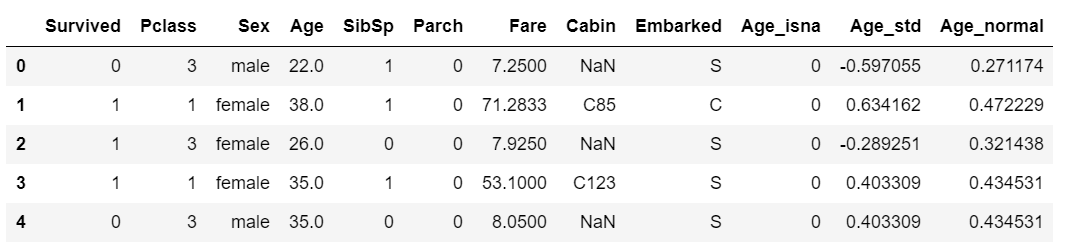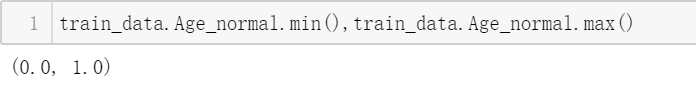（注意：标准化和归一化只选择其中一种方法即可）

• 多个变量之间的数学运算，例如构造一个新变量 z=a/b
• 基于逻辑判断来构造衍生变量，形如 SQL 中的 case when ... then ...这样的方式
• 多个特征变量进行组合，例如性别，年龄本来是两个独立的变量，可以把他们组合起来变成新的独 热编码变量：男&(0,18]，男&(18,25]...

SibSp和Parch数据

• SibSp：在船兄弟姐妹数/配偶数量
• Parch：在船父母数/子女数

``````# 构造新变量 FamilySize  （类别型变量）
train_data['FamilySize']=train_data['SibSp']+train_data['Parch']+1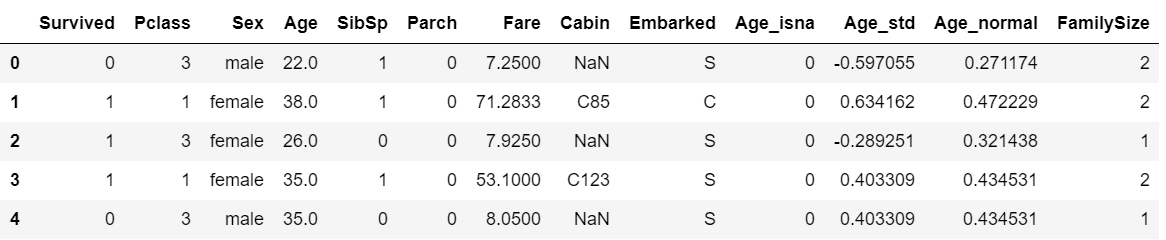通过前面使用过的分析方法来判断新的变量（FamilySize）与是否生还有一定的相关性。

``````# 使用交叉表分析FamilySize与Survived之间的相关性
pd.crosstab(train_data.Survived,train_data.FamilySize,margins=True)``````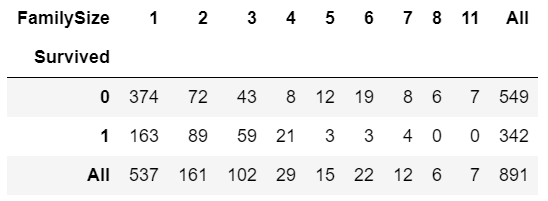``````# 按列统计交叉表百分比
# FamilySize与Survived的交叉表，显示列占比
pd.crosstab(train_data.Survived,train_data.FamilySize).apply(lambda r:r/r.sum(),axis=0)
``````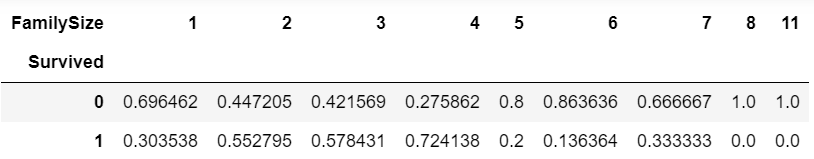1、构造一个新变量 IsAlone（是否是独自一个人登船)，判断逻辑：如果 FamilySize=1，那么 IsAlone=1，否则 IsAlone=0

``````train_data['IsAlone']=0     # 构造新的变量，赋值为0
train_data.loc[train_data['FamilySize']==1,'IsAlone']=1   # 变量FamilySize=1时，用1替换原来给IsAlone赋的值 0
train_data['IsAlone'].value_counts()  # 查看变量IsAlone的频数``````2、构造一个新变量 IsMother（是否是母亲），判断逻辑：如果性别是女性，同时父母/子女数大于 0，并且年龄大于 20 岁，那么 IsMother=1，否则 IsMother=0

``````train_data['IsMother'] = 0   # 构造新变量 IsMother，赋值为 0
train_data.loc[(train_data['Sex']=='female') & (train_data['Parch']>0) &(train_data['Age']>20),'IsMother'] = 1
train_data['IsMother'].value_counts()  # 频数统计``````多个特征变量的组合

``````#  对Sex和Age_bin进行组合,得到新的变量  Sex_Age_combo
train_data['Sex_Age_combo'] = train_data['Sex'] + "_" +train_data['Age_bin'].astype(str)
train_data['Sex_Age_combo'].value_counts()  # 新变量Sex_Age_combo 的频数``````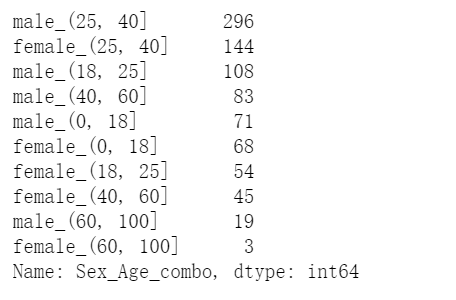``````# 对变量  Sex_Age_combo  进行独热编码
Sex_Age_combo_onehot=pd.get_dummies(train_data['Sex_Age_combo'],prefix='Sex_Age')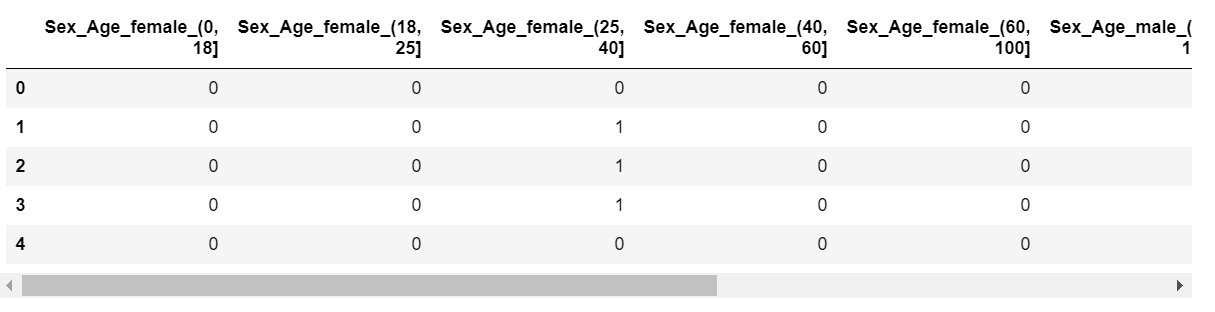``````# 把需要的变量拼接起来
TrainData=pd.concat([train_data[['Survived','Age_isna','IsAlone','IsMother']],Pclass,Sex,Embarked,AgeBin,FareBin,FamilySize,SexAgeCombo],axis=1)
TrainData.describe().transpose()  # 描述性统计``````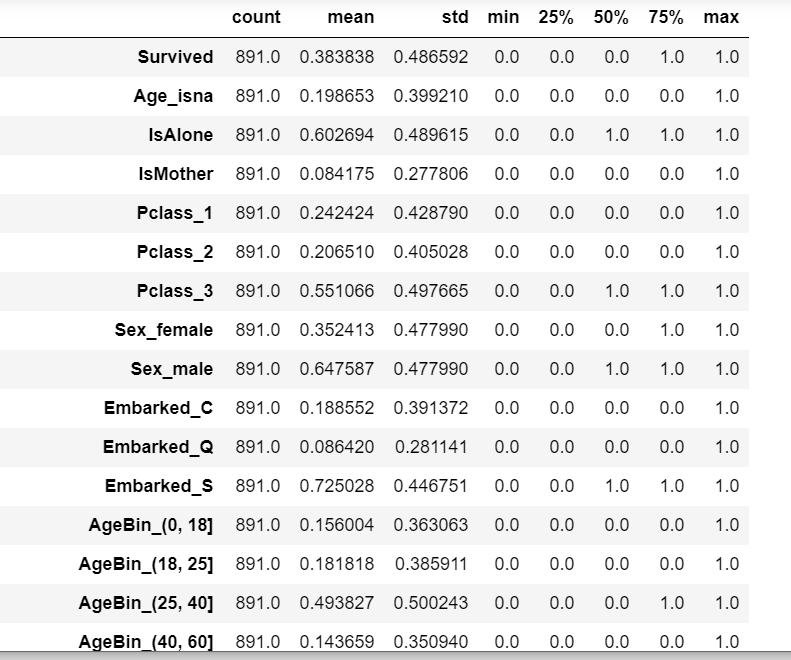``````TrainData.shape
# 运行结果：(891, 41)``````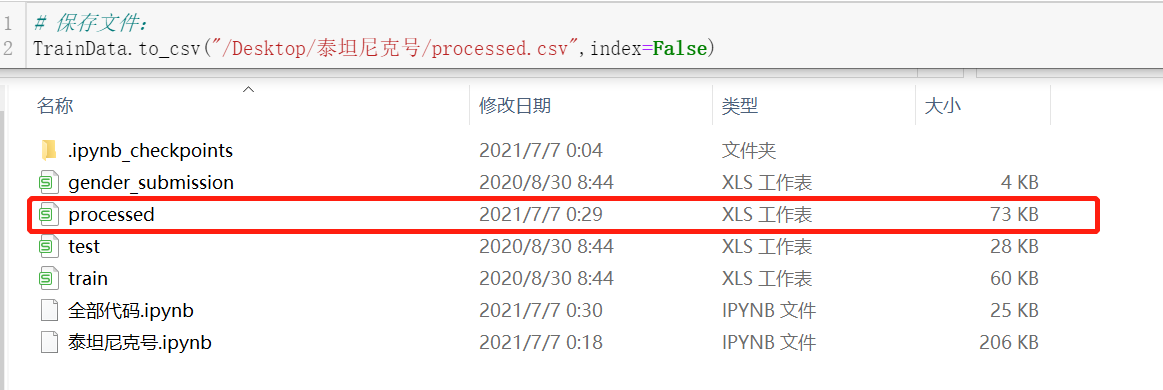``````trainData=pd.read_csv("Desktop\泰坦尼克号\processed.csv")
trainData_X=trainData.drop(['Survived'],axis=1)
trainData_y=trainData.Survived``````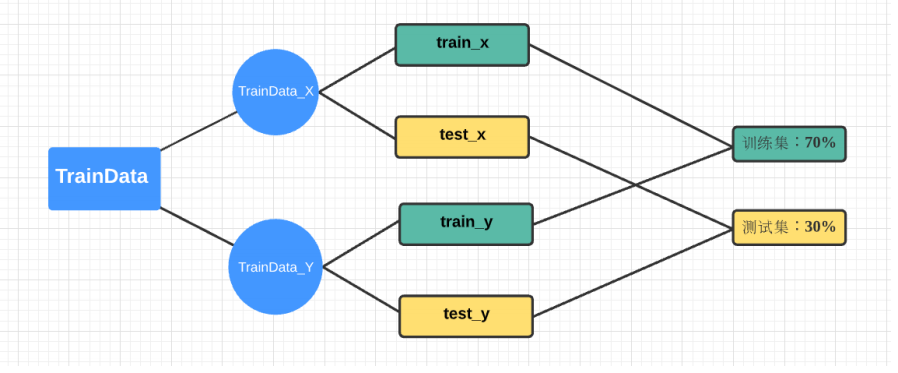train: 训练集数据          test:测试集数据

``````from sklearn.model_selection import train_test_split
train_x, test_x, train_y, test_y = train_test_split(trainData_X,trainData_y,test_size=0.3,random_state=123456)
``````

``````# 逻辑回归算法
from sklearn.linear_model import LogisticRegression
LR = LogisticRegression()
LR.fit(train_x, train_y)  # 训练集 （训练模型）

# 模型评估 （测试集）
from sklearn.metrics import classification_report
y_test_pred = LR.predict(test_x)     # y_test_pred是预测的结果
print(classification_report(test_y,y_test_pred))  # 评估模型（真实的值与预测的结果对比）``````